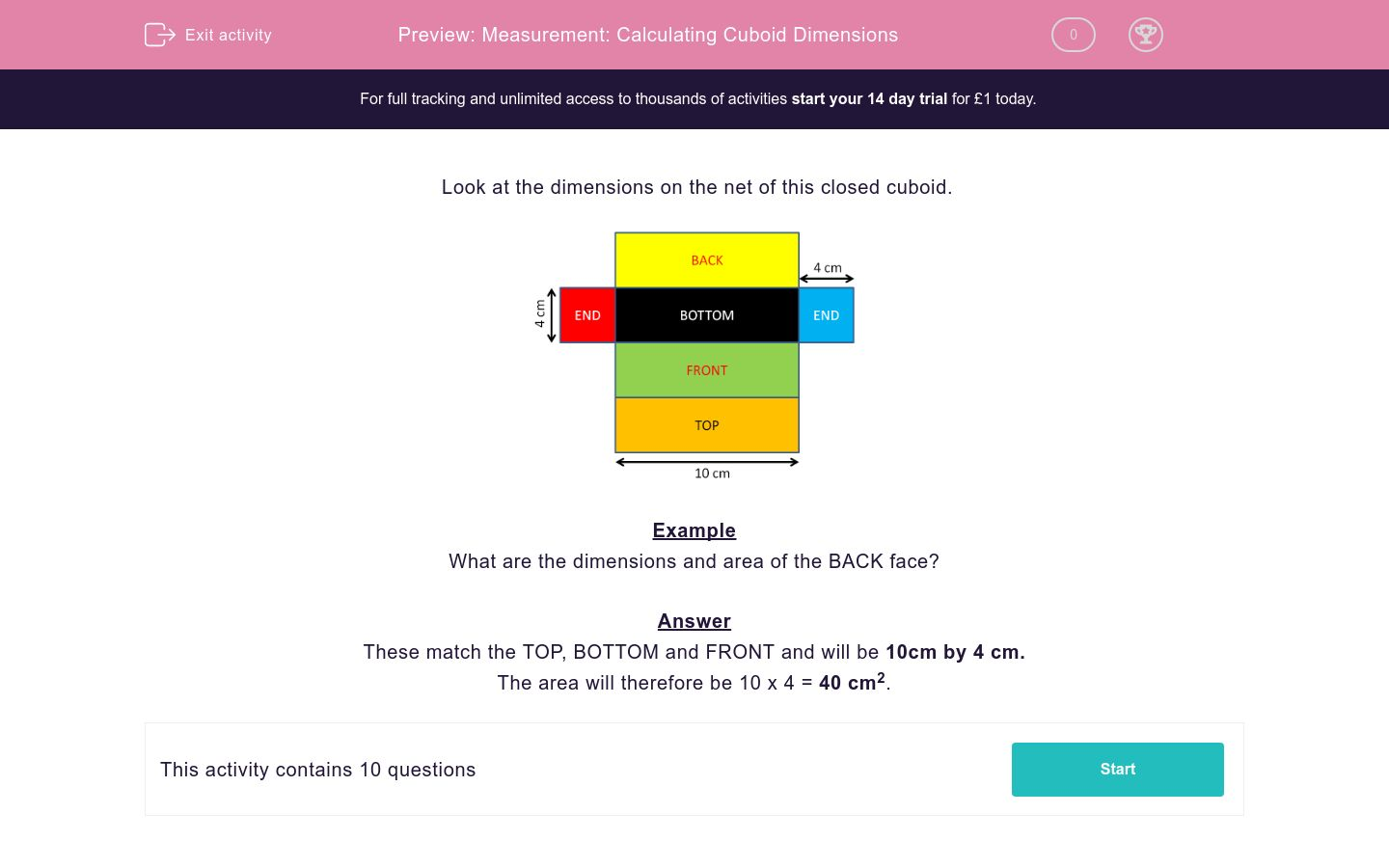# Measurement: Calculating Cuboid Dimensions

In this worksheet, students answer questions on the net of a cuboid.Key stage:  KS 2

Curriculum topic:   Maths and Numerical Reasoning

Curriculum subtopic:   Perimeter and Area

Difficulty level:### QUESTION 1 of 10

Look at the dimensions on the net of this closed cuboid.Example

What are the dimensions and area of the BACK face?

These match the TOP, BOTTOM and FRONT and will be 10cm by 4 cm.

The area will therefore be 10 x 4 = 40 cm2.

---- OR ----